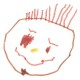# Using R to Produce Scalable Vector Graphics for the Web

Statistical software is normally used during the analysis stage of a project and a cleaned up static graphic is created for the presentation.  If the presentation is in web format then there are some considerations that are needed. The trick is to find ways to implement those graphs in that web format so the graph is of the highest possible quality.  If all that is needed is an image then simply saving the graph as a JPG or PNG and posting it to a website is quite simple and usually sufficient.  However, if more flexibility and higher quality is needed then some additional work will be needed.

One of the best way to present a graph is using vectors (as opposed to raster graphics).  What this means is that if one uses vectors graphics then a user can zoom in and there won’t be any degradation in image quality.  Several formats support vector graphics including PDF and SVG.

Scalable Vector Graphics are a great way to put together graphs using an XML-based format. This means it can be easily implemented directly into a website and, as an added bonus, it can become a dynamic image changing with user input.   R will generate the base structure of the graphic but dynamic SVG requires a bit more work outside of R.   Most modern browsers (IE 8 is not considered modern anymore so it is not supported) support this type of graphic format . Supported browsers include  IE 9, Firefox, and Chrome.

Using the example from a previous post I can convert the image into Scalable Vector Graphic.  The code that R produces into the SVG file can be copied and pasted directly into a web page. I have a side-by-side comparison of the graphs using earthquake data from the week prior to June 28, 2013.library(maps)
library(maptools)
library(rgdal)
svg(filename = “c:\\eq.svg” ,
width = 7, height = 7
)

plot.new()
my.map < - map("state", interior = FALSE, plot=F) x.lim <- my.map\$range[1:2]; x.lim <- x.lim-1; x.lim <- x.lim+1; y.lim <- my.map\$range[3:4]; y.lim <- y.lim-1; y.lim <- y.lim+1; map("state", interior = FALSE, xlim=x.lim, ylim=y.lim) map("state", boundary = FALSE, col="gray", add = TRUE) title("Magnitude 1+ Earthquakes Over the Past 7 Days") eq\$mag.size <- NULL eq\$mag.size[eq\$Magnitude>=1 & eq\$Magnitude < 2] < - .75 eq\$mag.size[eq\$Magnitude>=2 & eq\$Magnitude < 3] < - 1.0 eq\$mag.size[eq\$Magnitude>=3 & eq\$Magnitude < 4] < - 1.5 eq\$mag.size[eq\$Magnitude>=4] < - 2.0 eq\$mag.col <- NULL eq\$mag.col[eq\$Magnitude>=1 & eq\$Magnitude < 2] < - 'blue' eq\$mag.col[eq\$Magnitude>=2 & eq\$Magnitude < 3] < - 'green' eq\$mag.col[eq\$Magnitude>=3 & eq\$Magnitude < 4] < - 'orange' eq\$mag.col[eq\$Magnitude>=4] < - 'red' points(x=eq\$Lon,y=eq\$Lat,pch=16,cex=eq\$mag.size, col=eq\$mag.col) eq\$magnitude.text <- eq\$Magnitude eq\$magnitude.text[eq\$Magnitude < 4] <- NA text(x=eq\$Lon,y=eq\$Lat,col='black',labels=eq\$magnitude.text,adj=c(2.5),cex=0.5) legend('bottomright',c('M 1-2','M 2-3','M 3-4','M4+'), ncol=2, pch=16, col=c('blue','green','orange','red')) box() dev.off() [/sourcecode]   The package ggplot2 has a function that will identify that one wants an SVG file based on the filename provided.  This graph shows the depth of the earthquake compared to the magnitude.library(ggplot2)
( g <- ggplot(eq, aes(x = Magnitude, y = Depth)) + geom_point() + geom_point(data = eq, color = eq\$mag.col, size = 3) ) ggsave(g, file="c:\\eqDepthMagnitude.svg") [/sourcecode]

## 4 thoughts on “Using R to Produce Scalable Vector Graphics for the Web”

1.michael says:

Is it possible that you side by side comparison has the images reversed?

1.Wesley says:

In the side-by-side graph you will note that the image on the right is the scalable vector graphic. If you zoom in you will not see any degradation in that image quality. However, the image on the left (raster image) you can start to see the pixels as you zoom in.